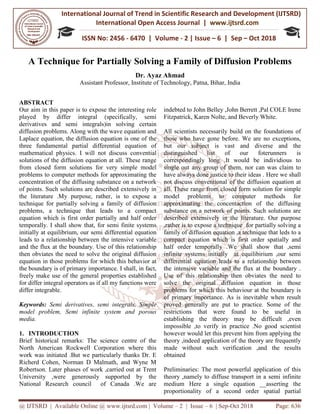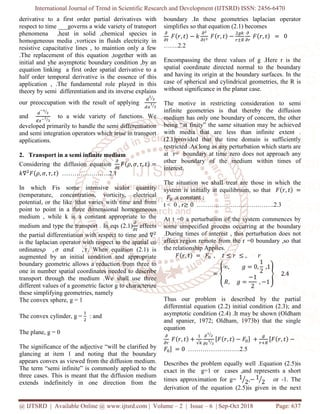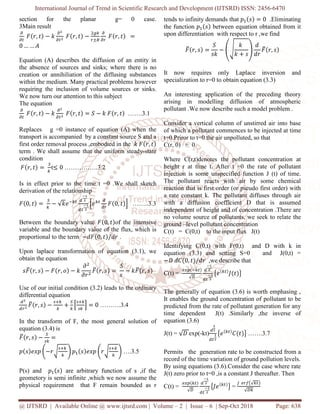Diese Präsentation wurde erfolgreich gemeldet.

# A Technique for Partially Solving a Family of Diffusion Problems

Anzeige
Anzeige
Anzeige
Anzeige
Anzeige
Anzeige
Anzeige
Anzeige
Anzeige
Anzeige
Anzeige×

1 von 4 Anzeige

# A Technique for Partially Solving a Family of Diffusion Problems

Our aim in this paper is to expose the interesting role played by differ integral specifically, semi derivatives and semi integrals in solving certain diffusion problems. Along with the wave equation and Laplace equation, the diffusion equation is one of the three fundamental partial differential equation of mathematical physics. I will not discuss convential solutions of the diffusion equation at all. These range from closed form solutions for very simple model problems to computer methods for approximating the concentration of the diffusing substance on a network of points. Such solutions are described extensively in the literature .My purpose, rather, is to expose a technique for partially solving a family of diffusion problems, a technique that leads to a compact equation which is first order partially and half order temporally. I shall show that, for semi finite systems initially at equilibrium, our semi differential equation leads to a relationship between the intensive variable and the flux at the boundary. Use of this relationship then obviates the need to solve the original diffusion equation in those problems for which this behavior at the boundary is of primary importance. I shall, in fact, freely make use of the general properties established for differ integral operators as if all my functions were differ integrable. Dr. Ayaz Ahmad "A Technique for Partially Solving a Family of Diffusion Problems" Published in International Journal of Trend in Scientific Research and Development (ijtsrd), ISSN: 2456-6470, Volume-2 | Issue-6 , October 2018, URL: http://www.ijtsrd.com/papers/ijtsrd18576.pdf

Our aim in this paper is to expose the interesting role played by differ integral specifically, semi derivatives and semi integrals in solving certain diffusion problems. Along with the wave equation and Laplace equation, the diffusion equation is one of the three fundamental partial differential equation of mathematical physics. I will not discuss convential solutions of the diffusion equation at all. These range from closed form solutions for very simple model problems to computer methods for approximating the concentration of the diffusing substance on a network of points. Such solutions are described extensively in the literature .My purpose, rather, is to expose a technique for partially solving a family of diffusion problems, a technique that leads to a compact equation which is first order partially and half order temporally. I shall show that, for semi finite systems initially at equilibrium, our semi differential equation leads to a relationship between the intensive variable and the flux at the boundary. Use of this relationship then obviates the need to solve the original diffusion equation in those problems for which this behavior at the boundary is of primary importance. I shall, in fact, freely make use of the general properties established for differ integral operators as if all my functions were differ integrable. Dr. Ayaz Ahmad "A Technique for Partially Solving a Family of Diffusion Problems" Published in International Journal of Trend in Scientific Research and Development (ijtsrd), ISSN: 2456-6470, Volume-2 | Issue-6 , October 2018, URL: http://www.ijtsrd.com/papers/ijtsrd18576.pdf

Anzeige
Anzeige